# Simplifying Expressions Algebraic Expressions Evaluating Expressions Technical Applications

• Slides: 12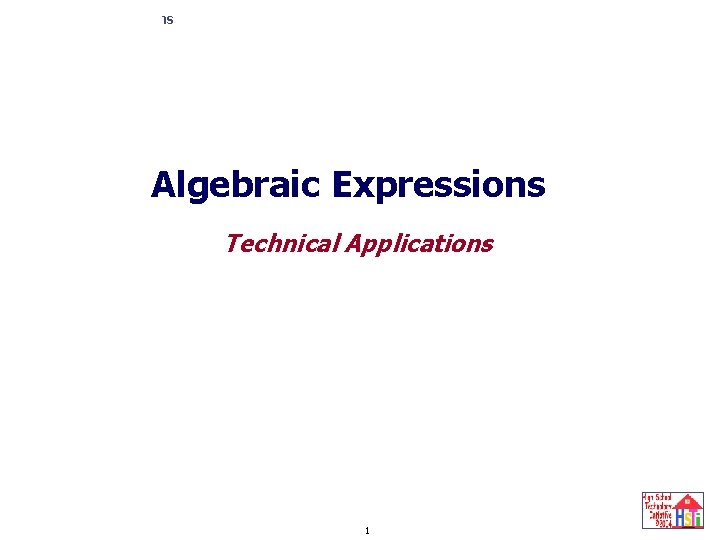Simplifying Expressions Algebraic Expressions Evaluating Expressions Technical Applications 1Simplifying Expressions Technical Application Scientists, engineers and technicians need, develop, and use mathematics to explain, describe, and predict what nature, processes, and equipment do. Many times the math they use is the math that is taught in ALGEBRA 1! 2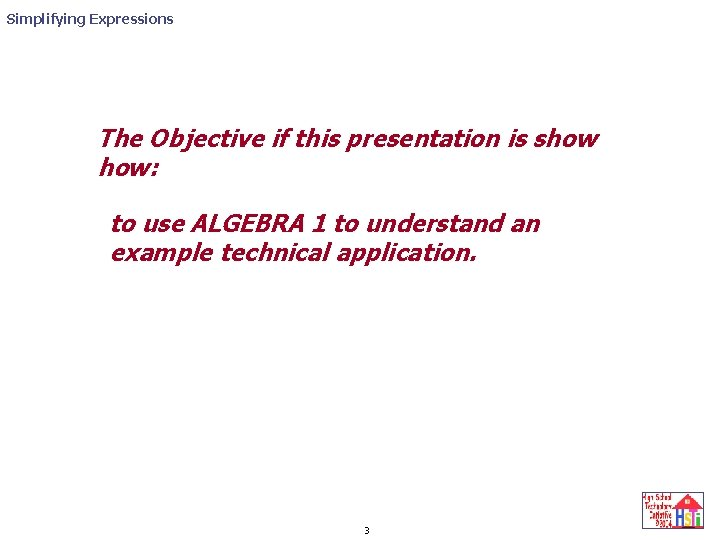Simplifying Expressions The Objective if this presentation is show how: to use ALGEBRA 1 to understand an example technical application. 3Simplifying Expressions Using Algebra in a Technical Application Consider the feasibility of using a hydrogen laser beam for application as a welding tool outside the space station. A laser beam is made when the an electron from many of the same type of atoms moves back from the same outer orbit to the same orbit closer to the atom’s nucleus. SIMPLIFIED LASER EXCITATION SOURCE Atoms emit light as photons after excitation LASER TUBE Some travel parallel to the laser tube and bounce off the mirrored ends Monochromatic light (LASER LIGHT) leaves the partially mirrored end Laser welding tool 4Simplifying Expressions Using Algebra in a Technical Application Consider the feasibility of using a hydrogen laser beam for application as a welding tool outside the space station. A laser beam is made when the an electron from many of the same type of atoms moves back from the same outer orbit to the same orbit closer to the atom’s nucleus. 2 st orbit out from nucleus (n 2) 3 nd orbit out from nucleus (n 3) A photon of light is emitted Atom’s nucleus If, for example, electrons move from 3 rd orbit to second orbit, a single color light will be emitted. 5 If the same electrons from enough of the same type of atoms go through this process, we may see a collection of light waves as a beam of a specific colored light.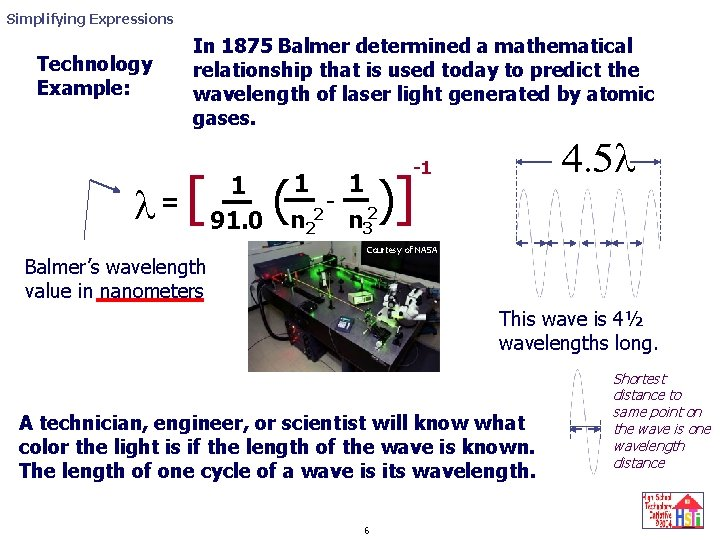Simplifying Expressions Technology Example: In 1875 Balmer determined a mathematical relationship that is used today to predict the wavelength of laser light generated by atomic gases. l= [ Balmer’s wavelength value in nanometers 1 91. 0 ( 4. 5 l -1 )] 1 1 - 2 2 n 3 Courtesy of NASA This wave is 4½ wavelengths long. A technician, engineer, or scientist will know what color the light is if the length of the wave is known. The length of one cycle of a wave is its wavelength. 6 Shortest distance to same point on the wave is one wavelength distanceSimplifying Expressions If the light beam for a hydrogen gas laser is to be generated when one electron from many individual hydrogen atoms in the gas move from the 3 rd orbit to the 2 nd orbit, what color is the light that is observed. Technology Application Problem Statement l [ ( = 1 91 Symbol people use to represent the wavelength value in nanometers -1 )] 1 1 - 1 2 2 2 3 91 )] =[ ( 1 1 - 2 2 n 3 l -1 -1 [ ( (4 9) )] (9 -4) =? 1 91 -1 -1 [ ( ) ] [ ( )] 0. 0015] = 670 nanometers [ = l l = 5 1 91 36 7 = -1 1 0. 14 91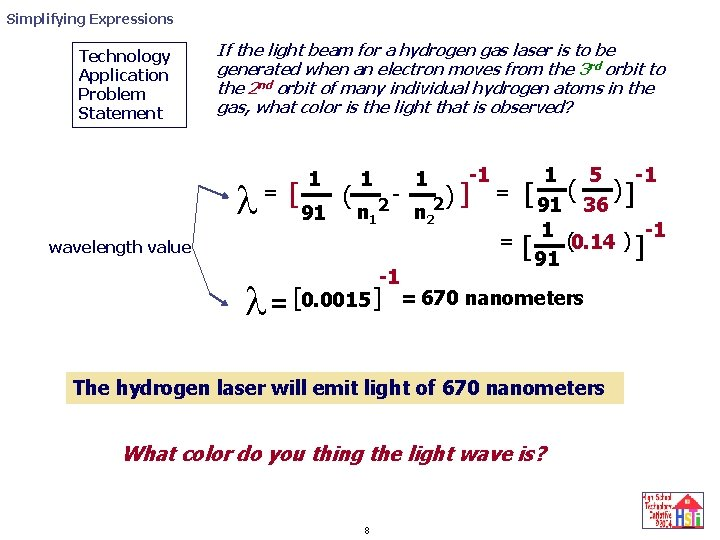Simplifying Expressions Technology Application Problem Statement If the light beam for a hydrogen gas laser is to be generated when an electron moves from the 3 rd orbit to the 2 nd orbit of many individual hydrogen atoms in the gas, what color is the light that is observed? l = [ 1 91 ( 1 n 1 2 - wavelength value -1 l = [0. 0015 ] 1 2) n 2 -1 ] 1 5 -1 ( ) = [ 91 36 ] 1 -1 = [ (0. 14 ) ] 91 = 670 nanometers The hydrogen laser will emit light of 670 nanometers What color do you thing the light wave is? 8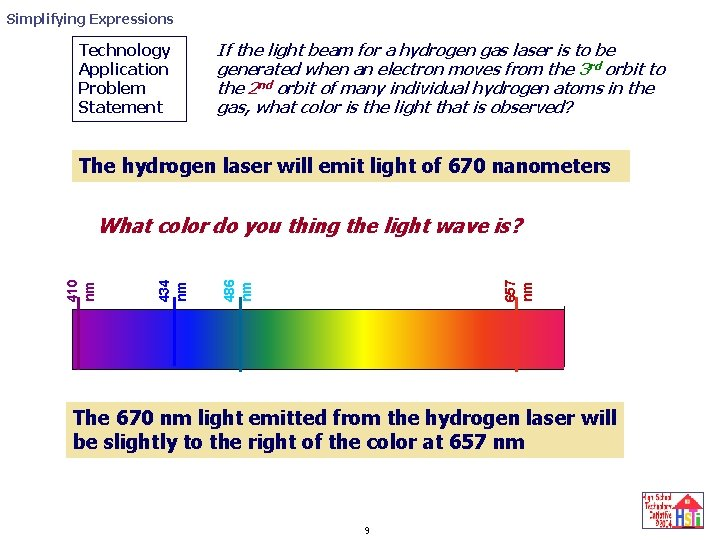Simplifying Expressions Technology Application Problem Statement If the light beam for a hydrogen gas laser is to be generated when an electron moves from the 3 rd orbit to the 2 nd orbit of many individual hydrogen atoms in the gas, what color is the light that is observed? The hydrogen laser will emit light of 670 nanometers 657 nm 486 nm 434 nm 410 nm What color do you thing the light wave is? The 670 nm light emitted from the hydrogen laser will be slightly to the right of the color at 657 nm 9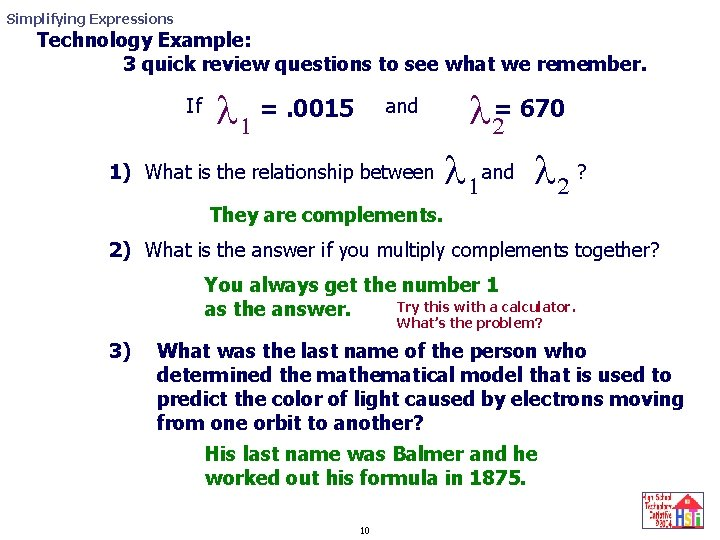Simplifying Expressions Technology Example: 3 quick review questions to see what we remember. l =2 670 What is the relationship between l and l ? 1 2 If 1) l 1 =. 0015 and They are complements. 2) What is the answer if you multiply complements together? You always get the number 1 Try this with a calculator. as the answer. What’s the problem? 3) What was the last name of the person who determined the mathematical model that is used to predict the color of light caused by electrons moving from one orbit to another? His last name was Balmer and he worked out his formula in 1875. 10Simplifying Expressions Technology Example: What do you think? 1) Do you think Balmer figured out his mathematical relationship so that he could understand the Bohr atomic model? Why/Why not? 2) Can you make a laser beam by focusing sun light through a prism? Why/ Why not? 3) Does the Balmer model predict that a laser beam will occur if electrons go from the 2 nd orbital to the first orbital of an atom? Why/why not. 11Simplifying Expressions 12